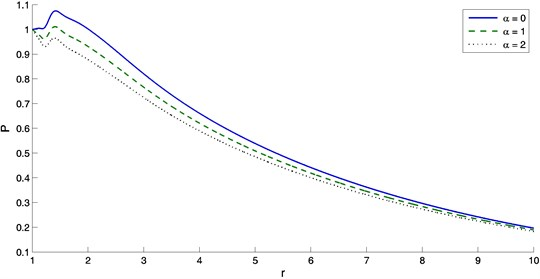Published: 31 December 2014

# The effect of thermal source with mass diffusion in a transversely isotropic thermoelastic infinite medium

Ibrahim A. Abbas1
1Mathematics Department, Faculty of Science and Arts – Khulais, King Abdulaziz University, Jeddah, Saudi Arabia
1Department of Mathematics, Faculty of Science, Sohag University, Sohag, Egypt
Views 136

#### Abstract

The theory of generalized thermoelastic diffusion with relaxation times, in the context of Lord and Shulman theory, is used to investigate the thermoelastic diffusion problem in a transversely isotropic thermoelastic infinite medium with a cylindrical cavity. The surface of cavity is taken to be traction free and subjected to heating. The analytical solution in the Laplace transform domain is obtained by using the eigenvalue approach. The numerical results of displacement, temperature, mass concentration and stress as well as the chemical potential represented analytical and graphically. An appreciable effect of relaxation times is observed on various resulting quantities.

## 1. Introduction

The generalized theory of thermoelasticity is one of the modified versions of the classical uncoupled and coupled theory of thermoelasticity and has been developed in order to remove the paradox of the physically impossible phenomena of infinite velocity of thermal signals in the classical coupled thermoelasticity. The first of such modeling is the extended thermoelasticity theory (LS) of Lord and Shulman , who introduced the concept of thermal relaxation time into the classical Fourier law of heat conduction. Subsequently, modifying the stress versus strain relationship as well as the entropy relationship with relaxation time, Green and Lindsay  proposed the temperature rate dependent thermoelasticity (GL) theory. The theory was extended for anisotropic body by Dhaliwal and Sherief . Recently, [4-13] have considered different problems by using the generalized thermoelasticity theories.

Diffusion can be defined as the random walk of an ensemble of particles, from regions of high concentration to regions of lower concentration. The studying of diffusion became increasingly important. This is due mainly to its many applications in geophysics and industrial applications. In integrated circuit fabrication, diffusion is used to introduce “dopants” in controlled amounts into the semiconductor substrate. In particular, diffusion is used to form the base and emitter in bipolar transistors, form integrated resistors, and form the source/drain regions in MOS transistors and dope poly-silicon gates in MOS transistors. The theory of thermoelastic diffusion is developed by [14-16]. In this theory, the coupled thermoelastic model is used.  developed the theory of generalized thermoelastic diffusion that predicts finite speeds of propagation for thermoelastic and diffusive waves. [18, 19] studied the theory of generalized thermoelastic diffusion in an infinite elastic body with a spherical cavity while [20, 21] investigated the diffusion in a generalized thermoelastic solid in an infinite body with a cylindrical cavity. The counterparts of our problem in the contexts of the thermoelasticity theories have been considered by using analytical and numerical methods [22-29].

In this paper, the thermoelastic interaction with mass diffusion in an infinite medium with a cylindrical cavity is investigated. Laplace transform techniques and eigenvalue approach are used. The physical explanations are given seriatim corresponding to the distributions of the considered physical variables obtained in this paper. An appreciable effect of relaxation times is observed on various resulting quantities.

## 2. Basic equations and problem formulation

Following Sherief et al.  and Abbas et al. , the governing equations for an anisotropic, homogeneous thermoelastic diffusion solid in the absence of body forces, heat source and mass diffusion source considered as:

– the equations of motion:

1
${c}_{ijkm}{e}_{km,j}-{a}_{ij}{T}_{,j}-{b}_{ij}{C}_{,j}=\rho \frac{{\partial }^{2}{u}_{i}}{\partial {t}^{2}},$

– the equation of heat conduction:

2
${K}_{ii}{T}_{,ii}=\left(\frac{\partial }{\partial t}+{\tau }_{o}\frac{{\partial }^{2}}{\partial {t}^{2}}\right)\left(\rho {c}_{e}T+{a}_{kk}{T}_{0}{e}_{kk}+a{T}_{0}C\right),$

– the equation of mass diffusion:

3
${D}_{ii}b{C}_{,ii}=\left(\frac{\partial }{\partial t}+{\tau }_{1}\frac{{\partial }^{2}}{\partial {t}^{2}}\right)C+{D}_{ii}{b}_{kk}{e}_{kk,ii}+{D}_{ii}a{T}_{,ii},$

– the constitutive equations:

4
${\sigma }_{ij}={c}_{ijkm}{e}_{km}-\left[{a}_{ij}\left(T-{T}_{0}\right)+{b}_{ij}C\right]{\delta }_{ij},$
5
$P=-{b}_{kk}{e}_{kk}+bC-a\left(T-{T}_{0}\right),$
6
${e}_{ij}=\frac{1}{2}\left({u}_{i,j}+{u}_{j,i}\right),$

where ${c}_{ijkm}$ are the elastic constants, $\rho$ is the density of the medium, ${u}_{i}$ are the components of displacement vector, $T$is the temperature, $C$ is the concentration of diffusive material in the elastic material, ${a}_{ij}$ and ${b}_{ij}$ are the tensor of thermal and diffusion moduli respectively and $t$ is the time. ${\tau }_{o}$ is the thermal relaxation time, ${K}_{ii}$ is the thermal conductivity, ${c}_{e}$ is the specific heat at constant strain, ${e}_{ij}$ are the components of strain tensor, ${T}_{0}$ is the reference temperature assumed to obey the inequality $\left|\frac{T-{T}_{0}}{{T}_{0}}\right|\ll 1$ and $a$ is the measures of thermodiffusion effect. ${\tau }_{1}$ is the diffusion relaxation time, ${D}_{ii}$ is the diffusion coefficient and $b$ is the measures of diffusive effects. ${\delta }_{ij}$ is the Kronecker symbol, ${\sigma }_{ij}$ are the components of stress tensor and $P$ is the chemical potential.

Let us consider an elastic unbounded body with a cylindrical cavity occupying the region $R\le r<\mathrm{\infty }$ whose state can be expressed in terms of the space variable $r$ and the time $t$. $\left(r,\theta ,z\right)$ are taken as the cylindrical coordinates with $z$-axis aligned along the cylinder axis. We note that due to symmetry, the only non-vanishing displacement component is the radial one ${u}_{r}=u\left(r,t\right)$. The strain tensor has the non-vanishing following components:

7
${e}_{rr}=\frac{\partial u}{\partial r},\mathrm{}\mathrm{}{e}_{\theta \theta }=\frac{u}{r}.$

Substituting for ${e}_{rr}$, ${e}_{\theta \theta }$ into equations (4) and (5) we get:

8
${\sigma }_{rr}={c}_{11}\frac{\partial u}{\partial r}+{c}_{12}\frac{u}{r}-{a}_{11}\left(T-{T}_{0}\right)-{b}_{11}C,$
9
${\sigma }_{\theta \theta }={c}_{12}\frac{\partial u}{\partial r}+{c}_{11}\frac{u}{r}-{a}_{11}\left(T-{T}_{0}\right)-{b}_{11}C,$
10
$P=-{b}_{11}\left(\frac{\partial u}{\partial r}+\frac{u}{r}\right)+bC-a\left(T-{T}_{0}\right),$

where ${a}_{11}=\left({c}_{11}+{c}_{12}\right){\alpha }_{t1}+{c}_{13}{\alpha }_{t3}$ and ${b}_{11}=\left({c}_{11}+{c}_{12}\right){\alpha }_{c1}+{c}_{13}{\alpha }_{c3}$, in which ${\alpha }_{t1}$, ${\alpha }_{t3}$ are the coefficients of linear thermal expansion along the radial and tangential directions while and ${\alpha }_{c1}$, ${\alpha }_{c3}$ are the coefficients of linear diffusion expansion along the radial and tangential directions. Thus, the equations (1), (2) and (3) can be written as:

11
${c}_{11}\frac{\partial e}{\partial r}-{a}_{11}\frac{\partial T}{\partial r}-{b}_{11}\frac{\partial C}{\partial r}=\rho \frac{{\partial }^{2}u}{\partial {t}^{2}},$
12
${K}_{11}\left(\frac{{\partial }^{2}T}{\partial {r}^{2}}+\frac{1}{r}\frac{\partial T}{\partial r}\right)=\left(\frac{\partial }{\partial t}+{\tau }_{o}\frac{{\partial }^{2}}{\partial {t}^{2}}\right)\left(\rho {c}_{e}T+{a}_{11}{T}_{0}e+a{T}_{0}C\right),$
13
${D}_{11}b\left(\frac{{\partial }^{2}C}{\partial {r}^{2}}+\frac{1}{r}\frac{\partial C}{\partial r}\right)=\left(\frac{\partial }{\partial t}+{\tau }_{1}\frac{{\partial }^{2}}{\partial {t}^{2}}\right)C+{D}_{11}{b}_{11}\left(\frac{{\partial }^{2}e}{\partial {r}^{2}}+\frac{1}{r}\frac{\partial e}{\partial r}\right)+{D}_{11}a\left(\frac{{\partial }^{2}T}{\partial {r}^{2}}+\frac{1}{r}\frac{\partial T}{\partial r}\right).$

Now, we introduce the following non-dimensional variables:

$\left({r}^{\mathrm{\text{'}}},{u}^{\mathrm{\text{'}}}\right)=c\omega \left(r,u\right),\mathrm{}\mathrm{}\mathrm{}\mathrm{}\left({t}^{\mathrm{\text{'}}},{{\tau }^{\mathrm{\text{'}}}}_{o},{{\tau }^{\mathrm{\text{'}}}}_{1}\right)=\omega {c}^{2}\left({t}^{\mathrm{\text{'}}},{{\tau }^{\mathrm{\text{'}}}}_{o},{{\tau }^{\mathrm{\text{'}}}}_{1}\right),\mathrm{}\mathrm{}\mathrm{}\mathrm{}{P}^{\mathrm{\text{'}}}=\frac{P}{{b}_{11}},$
$T\mathrm{\text{'}}=\frac{{a}_{11}\left(T-{T}_{0}\right)}{{c}_{11}},\mathrm{}\mathrm{}\mathrm{}\left({\sigma \mathrm{\text{'}}}_{rr},{\sigma \mathrm{\text{'}}}_{\theta \theta }\right)\mathrm{}=\frac{\left({\sigma }_{rr},{\sigma }_{\theta \theta }\right)}{{c}_{11}},\mathrm{}\mathrm{}\mathrm{}\mathrm{}C\mathrm{\text{'}}=\frac{{b}_{11}C}{{c}_{11}},$

where ${c}^{2}=\frac{{c}_{11}}{\rho }$ and $\omega =\frac{\rho {c}_{e}}{{K}_{11}}$.

Using the above dimensionless variables, the equations (8)-(13), take the form where we have dropped the primes for convenience:

14
$\frac{\partial e}{\partial r}-\frac{\partial T}{\partial r}-\frac{\partial C}{\partial r}=\frac{{\partial }^{2}u}{\partial {t}^{2}},$
15
$\frac{{\partial }^{2}T}{\partial {r}^{2}}+\frac{1}{r}\frac{\partial T}{\partial r}=\left(\frac{\partial }{\partial t}+{\tau }_{o}\frac{{\partial }^{2}}{\partial {t}^{2}}\right)\left(T+{\beta }_{u}e+{\beta }_{c}C\right),$
16
$\frac{{\partial }^{2}C}{\partial {r}^{2}}+\frac{1}{r}\frac{\partial C}{\partial r}={\psi }_{c}\left(\frac{\partial }{\partial t}+{\tau }_{1}\frac{{\partial }^{2}}{\partial {t}^{2}}\right)C+{\psi }_{u}\left(\frac{{\partial }^{2}e}{\partial {r}^{2}}+\frac{1}{r}\frac{\partial e}{\partial r}\right)+{\psi }_{t}\left(\frac{{\partial }^{2}T}{\partial {r}^{2}}+\frac{1}{r}\frac{\partial T}{\partial r}\right),$
17
${\sigma }_{rr}=\frac{\partial u}{\partial r}+\gamma \frac{u}{r}-T-C,$
18
${\sigma }_{\theta \theta }=\gamma \frac{\partial u}{\partial r}+\frac{u}{r}-T-C,$
19
$P={\chi }_{c}C-e-{\chi }_{t}T,$
20
$e=\frac{\partial u}{\partial r}+\frac{u}{r},$

where ${\beta }_{u}=\frac{{a}_{11}^{2}{T}_{0}}{\rho {c}_{e}{c}_{11}}$, ${\beta }_{c}=\frac{a{T}_{0}{a}_{11}}{\rho {c}_{e}{b}_{11}}$, $\gamma =\frac{{c}_{12}}{{c}_{11}}$, ${\psi }_{u}=\frac{{b}_{11}^{2}}{b{c}_{11}}$, ${\psi }_{c}=\frac{1}{b{D}_{11}\omega }$, ${\psi }_{t}=\frac{a{b}_{11}}{b{a}_{11}}$, ${\chi }_{c}=\frac{b{c}_{11}}{{b}_{11}^{2}}$, ${\chi }_{t}=\frac{a{c}_{11}}{{a}_{11}{b}_{11}}$.

The solution for the present problem is completed by the application of the initial and boundary conditions. The initial conditions can be expressed as:

21
$u\left(r,0\right)=\frac{\partial u\left(r,0\right)}{\partial t}=0,\mathrm{}\mathrm{}\mathrm{}T\left(r,0\right)=\frac{\partial T\left(r,0\right)}{\partial t}=0,\mathrm{}\mathrm{}\mathrm{}C\left(r,0\right)=\frac{\partial C\left(r,0\right)}{\partial t}=0.$

It is assumed that the inner surface of cavity is taken to be traction free and subject to a temperature which is decaying with time. The chemical potential is also assumed to be a known function of time on the inner surface of cavity. Therefore, the boundary conditions may be expressed as:

22
${\sigma }_{rr}\left(R,t\right)=0,\mathrm{}\mathrm{}\mathrm{}T\left(R,t\right)={T}_{1}{e}^{-\alpha t},\mathrm{}\mathrm{}\mathrm{}P\left(R,t\right)={P}_{1}H\left(t\right),\mathrm{}\mathrm{}\mathrm{}t>0,$

where ${T}_{1}$ and ${P}_{1}$ are constants, $\alpha$ is an exponent of the decayed heat flux and $H\left(t\right)$ denotes the Heaviside unit step function.

## 3. Solution in the Laplace transform domain

Applying the Laplace transform for above equations define by the formula:

23
$\stackrel{-}{f}\left(s\right)=L\left[f\left(t\right)\right]=\underset{0}{\overset{\mathrm{\infty }}{\int }}f\left(t\right){e}^{-st}dt.$

Hence, the equations (14)-(22) take the form:

24
$\frac{{d}^{2}\stackrel{-}{u}}{d{r}^{2}}+\frac{1}{r}\frac{d\stackrel{-}{u}}{dr}-\frac{\stackrel{-}{u}}{{r}^{2}}-\frac{d\stackrel{-}{T}}{dr}-\frac{d\stackrel{-}{C}}{dr}={s}^{2}\stackrel{-}{u},$
25
$\frac{{d}^{2}\stackrel{-}{T}}{d{r}^{2}}+\frac{1}{r}\frac{d\stackrel{-}{T}}{dr}=s\left(1+s{\tau }_{o}\right)\left(\stackrel{-}{T}+{\beta }_{u}\stackrel{-}{e}+{\beta }_{c}\stackrel{-}{C}\right),$
26
$\frac{{d}^{2}\stackrel{-}{C}}{d{r}^{2}}+\frac{1}{r}\frac{d\stackrel{-}{C}}{dr}={\psi }_{c}s\left(1+s{\tau }_{1}\right)\stackrel{-}{C}+{\psi }_{u}\left(\frac{{d}^{2}\stackrel{-}{e}}{d{r}^{2}}+\frac{1}{r}\frac{d\stackrel{-}{e}}{dr}\right)+{\psi }_{t}\left(\frac{{d}^{2}\stackrel{-}{T}}{d{r}^{2}}+\frac{1}{r}\frac{d\stackrel{-}{T}}{dr}\right),$
27
${\stackrel{-}{\sigma }}_{rr}=\frac{d\stackrel{-}{u}}{dr}+\gamma \frac{\stackrel{-}{u}}{r}-\stackrel{-}{T}-\stackrel{-}{C},$
28
${\stackrel{-}{\sigma }}_{\theta \theta }=\gamma \frac{d\stackrel{-}{u}}{dr}+\frac{\stackrel{-}{u}}{r}-\stackrel{-}{T}-\stackrel{-}{C},$
29
$\stackrel{-}{P}={\chi }_{c}\stackrel{-}{C}-\stackrel{-}{e}-{\chi }_{t}\stackrel{-}{T},$
30
$\stackrel{-}{e}=\frac{d\stackrel{-}{u}}{dr}+\frac{\stackrel{-}{u}}{r},$
31
${\stackrel{-}{\sigma }}_{rr}\left(R,s\right)=0,\mathrm{}\mathrm{}\mathrm{}\mathrm{}\stackrel{-}{T}\left(R,s\right)=\frac{{T}_{1}}{s+\alpha },\mathrm{}\mathrm{}\mathrm{}\mathrm{}\stackrel{-}{P}\left(R,s\right)=\frac{{P}_{1}}{s}.$

Differentiating equation (25) and (26) with respect to r and using equation (24) we get:

32
$\frac{{d}^{2}}{d\mathrm{}{r}^{2}}\left(\frac{d\stackrel{-}{T}}{dr}\right)+\frac{1}{r}\frac{d}{dr}\left(\frac{d\mathrm{}\stackrel{-}{T}}{dr}\right)-\frac{1}{{r}^{2}}\left(\frac{d\mathrm{}\stackrel{-}{T}}{dr}\right)={\zeta }_{1}\stackrel{-}{u}+{\zeta }_{2}\frac{d\mathrm{}\stackrel{-}{T}}{dr}+{\zeta }_{3}\frac{d\stackrel{-}{C}}{dr},$
33
$\frac{{d}^{2}}{d\mathrm{}{r}^{2}}\left(\frac{d\stackrel{-}{C}}{dr}\right)+\frac{1}{r}\frac{d}{dr}\left(\frac{d\mathrm{}\stackrel{-}{C}}{dr}\right)-\frac{1}{{r}^{2}}\left(\frac{d\mathrm{}\stackrel{-}{C}}{dr}\right)={\zeta }_{4}\stackrel{-}{u}+{\zeta }_{5}\frac{d\mathrm{}\stackrel{-}{T}}{dr}+{\zeta }_{6}\frac{d\stackrel{-}{C}}{dr},$

where ${\zeta }_{1}={s}^{2}{\beta }_{u}s\left(1+s{\tau }_{o}\right)\text{,}$${\zeta }_{2}=s\left(1+s{\tau }_{o}\right)\left(1+{\beta }_{u}\right)\text{,}$${\zeta }_{3}=s\left(1+s{\tau }_{o}\right)\left({\beta }_{u}+{\beta }_{c}\right)\text{,}$${\zeta }_{4}=\frac{{s}^{4}{\eta }_{u}+\left({\psi }_{u}+{\psi }_{t}\right){\zeta }_{1}}{1+{\psi }_{u}}\text{,}$${\zeta }_{5}=\frac{{s}^{2}{\psi }_{u}+\left({\psi }_{u}+{\psi }_{t}\right){\zeta }_{2}}{1+{\psi }_{u}}\text{,}$${\zeta }_{6}=\frac{s\left(1+s{\tau }_{1}\right){\psi }_{c}+{s}^{2}{\psi }_{u}+\left({\psi }_{u}+{\psi }_{t}\right){\zeta }_{3}}{1+{\psi }_{u}}$.

Equations (24), (32) and (33) can be written in a vector-matrix differential equation as follows :

34
$L\stackrel{\to }{V}=A\stackrel{\to }{V},$

where $L\equiv \frac{{d}^{2}}{d{r}^{2}}+\frac{1}{r}\frac{d}{dr}-\frac{1}{{r}^{2}}$, $\stackrel{\to }{V}={\left[\stackrel{-}{u}\mathrm{}\mathrm{}\stackrel{-}{T}\mathrm{}\mathrm{}\stackrel{-}{C}\mathrm{}\mathrm{}\frac{d\stackrel{-}{u}}{dr}\mathrm{}\mathrm{}\frac{d\stackrel{-}{T}}{dr}\mathrm{}\mathrm{}\frac{d\stackrel{-}{C}}{dr}\right]}^{T}$ and $A=\left[\begin{array}{lll}{s}^{2}& 1& 1\\ {\zeta }_{1}& {\zeta }_{2}& {\zeta }_{3}\\ {\zeta }_{4}& {\zeta }_{5}& {\zeta }_{6}\end{array}\right]$.

## 4. Solution of the vector-matrix differential equation

Let us now proceed to solve equation (34) by the eigenvalue approach proposed by . The characteristic equation of the matrix $B$ takes the form:

35
${\lambda }^{3}+{\lambda }^{2}\left(-{\zeta }_{2}-{\zeta }_{6}-{s}^{2}\right)+\lambda \left({\zeta }_{6}{s}^{2}-{\zeta }_{1}-{\zeta }_{4}-{\zeta }_{3}{\zeta }_{5}+{\zeta }_{2}{\zeta }_{6}+{\zeta }_{2}{s}^{2}\right)$
$+{\zeta }_{2}{\zeta }_{4}-{\zeta }_{3}{\zeta }_{4}-{\zeta }_{1}{\zeta }_{5}+{\zeta }_{1}{\zeta }_{6}+{\zeta }_{3}{\zeta }_{5}{s}^{2}-{\zeta }_{2}{\zeta }_{6}{s}^{2}=0.$

The roots of the characteristic equation (34) which are also the eigenvalues of matrix $A$ may be written in the form:

36
$\lambda ={\lambda }_{1},\mathrm{}\mathrm{}\lambda ={\lambda }_{2},\mathrm{}\mathrm{}\lambda ={\lambda }_{3}.$

The eigenvector $\stackrel{\to }{X}={\left[{X}_{1},{X}_{2},{X}_{3}\right]}^{T}$, corresponding to eigenvalue $\lambda$ can be calculated as:

37
${X}_{1}={\zeta }_{2}-{\zeta }_{3}-\lambda ,$
38
${X}_{2}=-{\zeta }_{1}-{\zeta }_{3}\left(\lambda -{s}^{2}\right),$
39
${X}_{3}={\zeta }_{1}+\left({\zeta }_{2}-\lambda \right)\left(\lambda -{s}^{2}\right).$

From equations (36)-(39), we can easily calculate the eigenvector ${\stackrel{\to }{X}}_{j}$, corresponding to eigenvalue ${\xi }_{j}$, $j=1,2,3$. For further reference, we shall use the following notations:

40
${\stackrel{\to }{X}}_{1}={\left[\stackrel{\to }{X}\right]}_{\lambda ={\lambda }_{1}},\mathrm{}\mathrm{}\mathrm{}\mathrm{}\mathrm{}{\stackrel{\to }{X}}_{2}={\left[\stackrel{\to }{X}\right]}_{\lambda ={\lambda }_{2}},\mathrm{}\mathrm{}\mathrm{}\mathrm{}\mathrm{}{\stackrel{\to }{X}}_{3}={\left[\stackrel{\to }{X}\right]}_{\lambda ={\lambda }_{3}}.$

The solution of equation (34) which is bounded as $r\to \mathrm{\infty }$, is given by:

41
$\stackrel{\to }{V}={\stackrel{\to }{X}}_{1}{B}_{1}{K}_{1}\left({m}_{1}r\right)+{\stackrel{\to }{X}}_{2}{B}_{2}{K}_{1}\left({m}_{2}r\right)+{\stackrel{\to }{X}}_{3}{B}_{3}{K}_{1}\left({m}_{3}r\right),$

where ${m}_{1}=\sqrt{{\lambda }_{1}}$, ${m}_{2}=\sqrt{{\lambda }_{2}}$, ${m}_{3}=\sqrt{{\lambda }_{3}}$, ${K}_{1}$ is the modified of Bessel’s function of order one, ${B}_{1}$, ${B}_{2}$ and ${B}_{3}$ are constants to be determined from the boundary condition of the problem. Thus, the field variables can be written for $r$ and $s$ as:

42
$\stackrel{-}{u}\left(r,\mathrm{s}\right)={x}_{1}^{1}{B}_{1}{K}_{1}\left({m}_{1}r\right)+{x}_{1}^{2}{B}_{2}{K}_{1}\left({m}_{2}r\right)+{x}_{1}^{3}{B}_{3}{K}_{1}\left({m}_{3}r\right),$
43
$\stackrel{-}{T}\left(r,s\right)=-\frac{{x}_{2}^{1}}{{m}_{1}}{B}_{1}{K}_{0}\left({m}_{1}r\right)-\frac{{x}_{2}^{2}}{{m}_{2}}{B}_{1}{K}_{0}\left({m}_{2}r\right)-\frac{{x}_{2}^{3}}{{m}_{3}}{B}_{3}{K}_{0}\left({m}_{3}r\right),$
44
$\stackrel{-}{C}\left(r,s\right)=-\frac{{x}_{3}^{1}}{{m}_{1}}{B}_{1}{K}_{0}\left({m}_{1}r\right)-\frac{{x}_{3}^{2}}{{m}_{2}}{B}_{1}{K}_{0}\left({m}_{2}r\right)-\frac{{x}_{3}^{3}}{{m}_{3}}{B}_{3}{K}_{0}\left({m}_{3}r\right),$
45
${\stackrel{-}{\sigma }}_{rr}\left(r,s\right)={B}_{1}\left(\frac{\left(-{m}_{1}^{2}{x}_{1}^{1}+{x}_{2}^{1}+{x}_{3}^{1}\right){K}_{0}\left({m}_{1}r\right)}{{m}_{1}}+\frac{\left(\eta -1\right){x}_{1}^{1}{K}_{1}\left({m}_{1}r\right)}{r}\right)$
$+{B}_{2}\left(\frac{\left(-{m}_{2}^{2}{x}_{1}^{2}+{x}_{2}^{2}+{x}_{3}^{2}\right){K}_{0}\left({m}_{2}r\right)}{{m}_{2}}+\frac{\left(\eta -1\right){x}_{1}^{2}{K}_{1}\left({m}_{2}r\right)}{r}\right)$
$+{B}_{3}\left(\frac{\left(-{m}_{3}^{2}{x}_{1}^{3}+{x}_{2}^{3}+{x}_{3}^{3}\right){K}_{0}\left({m}_{3}r\right)}{{m}_{3}}+\frac{\left(\eta -1\right){x}_{1}^{3}{K}_{1}\left({m}_{3}r\right)}{r}\right),$
46
${\stackrel{-}{\sigma }}_{\theta \theta }\left(r,s\right)={B}_{1}\left(\frac{\left(-\eta {m}_{1}^{2}{x}_{1}^{1}+{x}_{2}^{1}+{x}_{3}^{1}\right){K}_{0}\left({m}_{1}r\right)}{{m}_{1}}-\frac{\left(\eta -1\right){x}_{1}^{1}{K}_{1}\left({m}_{1}r\right)}{r}\right)$
$+{B}_{2}\left(\frac{\left(-\eta {m}_{2}^{2}{x}_{1}^{2}+{x}_{2}^{2}+{x}_{3}^{2}\right){K}_{0}\left({m}_{2}r\right)}{{m}_{2}}-\frac{\left(\eta -1\right){x}_{1}^{2}{K}_{1}\left({m}_{2}r\right)}{r}\right)$
$+{B}_{3}\left(\frac{\left(-\eta {m}_{3}^{2}{x}_{1}^{3}+{x}_{2}^{3}+{x}_{3}^{3}\right){K}_{0}\left({m}_{3}r\right)}{{m}_{3}}-\frac{\left(\eta -1\right){x}_{1}^{3}{K}_{1}\left({m}_{3}r\right)}{r}\right),$
47
$\stackrel{-}{P}\left(r,s\right)=\frac{{B}_{1}}{{m}_{1}}\left(-{\zeta }_{c}{x}_{3}^{1}+{m}_{1}^{2}{x}_{1}^{1}+{\zeta }_{t}{x}_{2}^{1}\right){K}_{0}\left({m}_{1}r\right)+\mathrm{}\frac{{B}_{2}}{{m}_{2}}\left(-{\zeta }_{c}{x}_{3}^{2}+{m}_{2}^{2}{x}_{1}^{2}+{\zeta }_{t}{x}_{2}^{2}\right){K}_{0}\left({m}_{2}r\right)$
$+\frac{{B}_{3}}{{m}_{3}}\left(-{\zeta }_{c}{x}_{3}^{3}+{m}_{3}^{2}{x}_{1}^{3}+{\zeta }_{t}{x}_{2}^{3}\right){K}_{0}\left({m}_{3}r\right).$

To complete the solution we have to know the constants ${B}_{1}$, ${B}_{2}$ and ${B}_{3}$ by using the boundary conditions (31) we can obtain:

48
$\left(\begin{array}{l}{B}_{1}\\ {B}_{2}\\ {B}_{3}\end{array}\right)={\left(\begin{array}{lll}{H}_{11}& {H}_{12}& {H}_{13}\\ {H}_{21}& {H}_{22}& {H}_{23}\\ {H}_{31}& {H}_{32}& {H}_{33}\end{array}\right)}^{-1}\left(\begin{array}{c}0\\ \frac{{T}_{1}}{s+\alpha }\\ \frac{{P}_{1}}{s}\end{array}\right),$

where:

${H}_{11}=\frac{\left(-{m}_{1}^{2}{x}_{1}^{1}+{x}_{2}^{1}+{x}_{3}^{1}\right){K}_{0}\left({m}_{1}R\right)}{{m}_{1}}+\frac{\left(\eta -1\right){x}_{1}^{1}{K}_{1}\left({m}_{1}R\right)}{R},$
${H}_{12}=\frac{\left(-{m}_{2}^{2}{x}_{1}^{2}+{x}_{2}^{2}+{x}_{3}^{2}\right){K}_{0}\left({m}_{2}R\right)}{{m}_{2}}+\frac{\left(\eta -1\right){x}_{1}^{2}{K}_{1}\left({m}_{2}R\right)}{R},$
${H}_{13}=\frac{\left(-{m}_{3}^{2}{x}_{1}^{3}+{x}_{2}^{3}+{x}_{3}^{3}\right){K}_{0}\left({m}_{3}R\right)}{{m}_{3}}+\frac{\left(\eta -1\right){x}_{1}^{3}{K}_{1}\left({m}_{3}R\right)}{R},$
$\begin{array}{lll}{H}_{21}=-\frac{{x}_{2}^{1}}{{m}_{1}}{B}_{1}{K}_{0}\left({m}_{1}R\right),& {H}_{22}=-\frac{{x}_{2}^{2}}{{m}_{2}}{B}_{1}{K}_{0}\left({m}_{2}R\right),& {H}_{23}=-\frac{{x}_{2}^{3}}{{m}_{3}}{B}_{3}{K}_{0}\left({m}_{3}R\right),\end{array}$
${H}_{31}=\frac{{B}_{1}}{{m}_{1}}\left(-{\zeta }_{c}{x}_{3}^{1}+{m}_{1}^{2}{x}_{1}^{1}+{\zeta }_{t}{x}_{2}^{1}\right){K}_{0}\left({m}_{1}R\right),$
${H}_{32}=\mathrm{}\frac{{B}_{2}}{{m}_{2}}\left(-{\zeta }_{c}{x}_{3}^{2}+{m}_{2}^{2}{x}_{1}^{2}+{\zeta }_{t}{x}_{2}^{2}\right){K}_{0}\left({m}_{2}R\right),$
${H}_{33}=\frac{{B}_{3}}{{m}_{3}}\left(-{\zeta }_{c}{x}_{3}^{3}+{m}_{3}^{2}{x}_{1}^{3}+{\zeta }_{t}{x}_{2}^{3}\right){K}_{0}\left({m}_{3}R\right).$

## 5. Numerical inversion of the Laplace transform

In order to determine the conductive and thermal temperature, displacement and stress distributions in the time domain, the Riemann-sum approximation method is used to obtain the numerical results. In this method, any function in Laplace domain can be inverted to the time domain as:

49
$f\left(t\right)=\frac{{e}^{\kappa t}}{t}\left[\frac{1}{2}\stackrel{-}{f}\left(\epsilon \right)+\mathrm{R}\mathrm{e}\sum _{n=1}^{N}{\left(-1\right)}^{n}\stackrel{-}{f}\left(\epsilon +\frac{i\mathrm{}n\pi }{t}\right)\right],$

where $\mathrm{R}\mathrm{e}$ is the real part and $i$ is imaginary number unit. For faster convergence, numerous numerical experiments have shown that the value of $\epsilon$ satisfies the relation $\epsilon t\approx 4.7$ .

## 6. Numerical results and discussion

The material was chosen for purposes of numerical evaluations and the constants of the problem were taken as following :

${c}_{11}=18.78×1{0}^{10}\frac{\mathrm{K}\mathrm{g}}{\mathrm{m}\bullet {\mathrm{s}}^{2}},\mathrm{}\mathrm{}\mathrm{}{c}_{12}=8.76×1{0}^{10}\frac{\mathrm{K}\mathrm{g}}{\mathrm{m}\bullet {\mathrm{s}}^{2}},\mathrm{}\mathrm{}\mathrm{}{c}_{13}=8.0×1{0}^{10}\frac{\mathrm{K}\mathrm{g}}{\mathrm{m}\bullet {\mathrm{s}}^{2}},$
${c}_{33}=18.2×1{0}^{10}\frac{\mathrm{K}\mathrm{g}}{\mathrm{m}\bullet {\mathrm{s}}^{2}},\mathrm{}\mathrm{}\mathrm{}{c}_{44}=5.06×1{0}^{10}\frac{\mathrm{K}\mathrm{g}}{\mathrm{m}\bullet {\mathrm{s}}^{2}},\mathrm{}\mathrm{}\mathrm{}{T}_{0}=.293×1{0}^{3}\mathrm{}\mathrm{K},$
${\alpha }_{1}=2.98×1{0}^{-5}{\mathrm{K}}^{-1},\mathrm{}\mathrm{}\mathrm{}{\alpha }_{3}=2.4×1{0}^{-5}{\mathrm{K}}^{-1},\mathrm{}\mathrm{}\mathrm{}\mathrm{}{\alpha }_{1c}=2.1×1{0}^{-4}{\mathrm{m}}^{3},$
${\alpha }_{3c}=2.5×1{0}^{-4}\frac{{\mathrm{m}}^{3}}{\mathrm{K}\mathrm{g}},\mathrm{}\mathrm{}\mathrm{}a=2.4×1{0}^{4}\frac{{\mathrm{m}}^{2}}{{\mathrm{s}}^{2}\mathrm{K}\mathrm{g}},\mathrm{}\mathrm{}\mathrm{}b=13.2×\mathrm{}1{0}^{5}\frac{{\mathrm{m}}^{5}}{{\mathrm{s}}^{2}\mathrm{K}\mathrm{g}},$
$\rho =8.954×1{0}^{3}\frac{\mathrm{K}\mathrm{g}}{{\mathrm{m}}^{3}},\mathrm{}\mathrm{}\mathrm{}{K}_{11}=0.433×1{0}^{3}\frac{\mathrm{W}}{\mathrm{m}\bullet \mathrm{K}},\mathrm{}\mathrm{}\mathrm{}{K}_{33}=0.450×1{0}^{3}\frac{\mathrm{W}}{\mathrm{m}\bullet \mathrm{K}},$
${D}_{11}=0.95×1{0}^{-8}\mathrm{}\frac{\mathrm{K}\mathrm{g}\bullet \mathrm{s}}{{\mathrm{m}}^{3}},$
$t=0.3,\mathrm{}\mathrm{}R=1,\mathrm{}\mathrm{}{T}_{1}=1,\mathrm{}\mathrm{}{\tau }_{o}=0.01,\mathrm{}\mathrm{}{\tau }_{1}=0.02,\mathrm{}\mathrm{}{p}_{1}=1.$

The effect of the exponent of the decayed heat flux $\alpha$ on the distribution of all quantities through the thickness of the medium is investigated. The numerical technique outlined above was used to obtain the temperature, displacement, concentration, radial stress, hoop stress and the chemical potential distributions respect to distance $r$ at $t=0.3$ for different values of the decayed heat flux $\alpha$. Figure 1 show the temperature decreases with the increase of the distance. It is noticed that the temperature always starts from different certain values according to the value of $\alpha$ and terminates at the zero value. Of course, $T$ decreases with the increase of the exponent of the decayed heat flux $\alpha$. It is obvious from figure 2 that the displacement is negative at $r=R$ where its magnitude is maximum. The displacement increases from the negative value to a positive value. In the positive values, the displacement has a peak value that depends on the values of the decayed heat flux $\alpha$. Figure 3 show the distribution of concentration with distance $r$. It is noticed that the concentration have a maximum magnitude at the boundary then decreases with the increasing of distance $r$ while it increase when increasing the decayed heat flux $\alpha$. Figure 4 display the variation of radial stress with distance $r$ for different values of $\alpha$. Its magnitude increases from zero to a maximum value after that decreases rapidly as $r$ increases and then the stress reduces to zero which agree with our theoretical boundary condition. Figure 5 display the variation of the hoop stress with the distance $r$ for different values of $\alpha$. Figure 6 show the variation of chemical potential with the distance $r$. From figure 6, it is seen that the chemical potential starts with its maximum value, which satisfies the boundary conditions and decreases with the increasing of the distance $r$.

Fig. 1The variation of temperature with distance r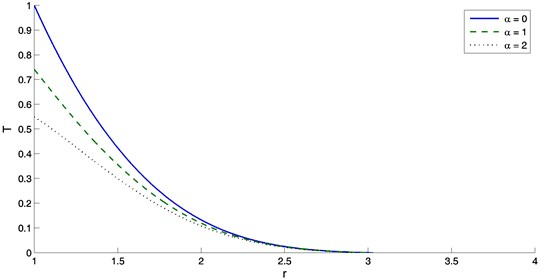Fig. 2The variation of displacement with distance r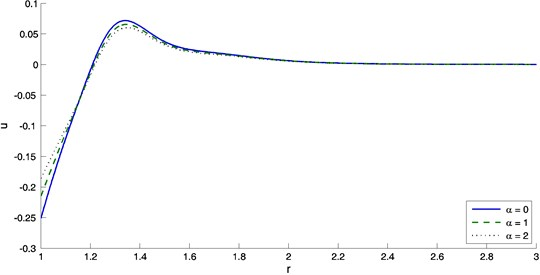Fig. 3The variation of concentration with distance r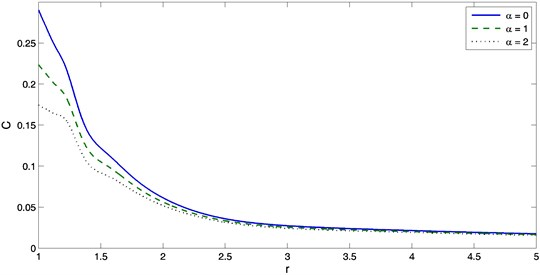Fig. 4The variation of radial stress with distance r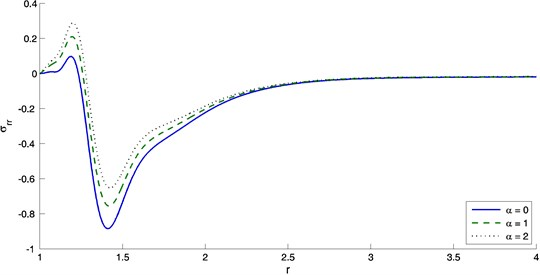Fig. 5The variation of hoop stress with distance r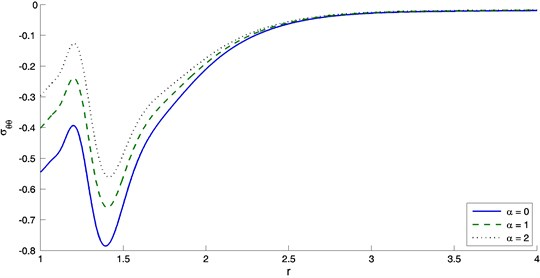Fig. 6The variation of chemical potential with distance r